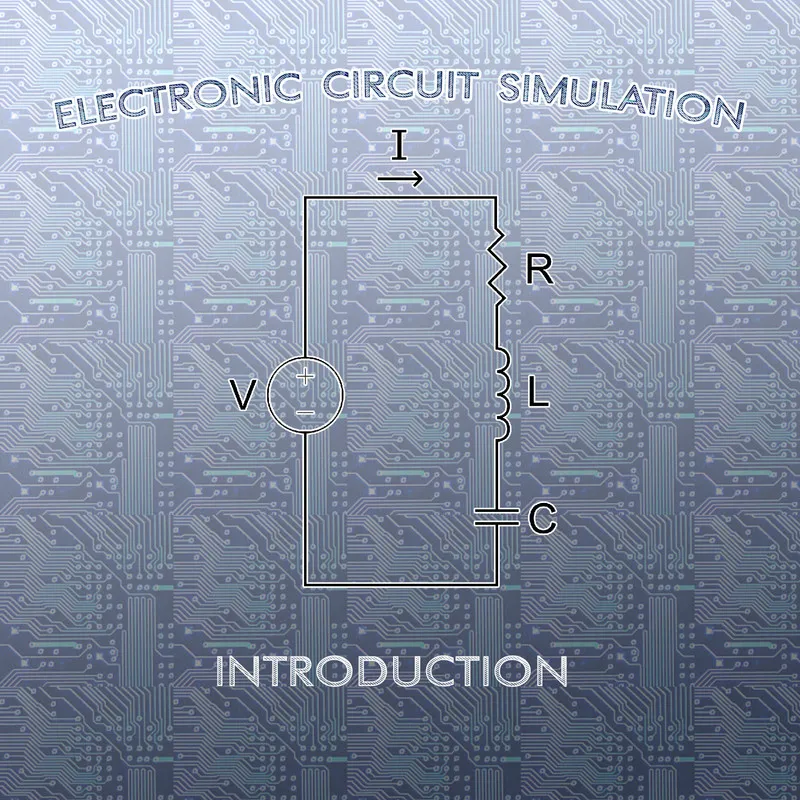# Electronic Circuit Simulation - Introduction [Python][Custom Thumbnail]

All the Code of the series can be found at the Github repository:
https://github.com/drifter1/circuitsim

# Introduction

Hello it's a me again @drifter1! Today we continue with the Electric Circuit Simulation series, a tutorial series where we will be implementing a full-on electronic circuit simulator (like SPICE) studying the whole concept and mainly physics behind it! This article is a quick introduction of what this series is all about.

## Requirements:

• Physics and more specifically Electromagnetism Knowledge
• Knowing how to solve Linear Systems using Linear Algebra
• Some understanding of the Programming Language Python

## Difficulty:

Talking about the series in general this series can be rated:
Today's topic(s) can be rated:
• Basic

So, without further ado, let's now get into the Series Description...

# Actual Tutorial Content

## Requirements

I already briefly explained the Requirements, as they will always occur in the beginning of articles, but let's get more specific! Talking about Electronic Circuit Simulation, someone can easily understand why Electromagnetism Physics are important!

### Electromagnetism

For the sake of this series, somebody will be fine, if he has an understanding of the following quantities:
• Electric Charge (Q)
• Electric Current (I)
• Electric Voltage or Potential Difference (V)
• Electric Resistance (R) and Conductance (G)
• Electric Capacitance (C) and Inductance (L)

A quite important law of Electromagnetism is Ohm's law, that I guess anybody learned in school. Of course this law will be used in lots of calculations, to substitute current, voltage or resistance. We should also don't forget Kirchhoff's laws which will be quite useful in the Matrix Analysis Techniques that we will be doing!

Talking about Electronic Circuits, those are build up of Electric Components, that can be Passive and Active, and so you should be able to distinguish these two categories. Furthermore, you should also be able to understand the difference between nodes and junctions in an electronic circuit.

### Solving Linear Systems

Understanding how to solve linear systems will be quite useful for you, as simple Linear Algebra and more complicated techniques can be used to solve the final linear system that we end up with after the whole Matrix Analysis procedure. But, as you might have guessed already, using Python (or even other languages) there are tools and libraries that do the solving for us and so in the end we will just have to call a function to solve the linear system for us! Either way, some understanding will be helpful.

### Python Language

And don't know how well all of you are aware of the Language "Python". It's an object-oriented language that has a quite simple syntax that makes writing much simpler. Code in this language looks quite similar to pseudo code of algorithms and so someone without any knowledge of this language could use this opportunity to learn this language by using it for this specific application of Electronic Circuit Simulation! I will explain every single line of code in-depth (it's a tutorial for some reason!) and so don't worry, be happy!😊

## Description

So, how will this series start? Well, even if you already have knowledge around Electromagnetism, a quick setup for the more complicated Matrix Analysis Techniques can be quite useful! This means that this series will start with a quick refreshment of the Required Electromagnetism Knowledge, split up in 4 articles, where we will cover:
• Electric Charge, Current, Field, Voltage (Potential Difference), Power, Wattage
• Electric Resistance and Conductance, Ohm's Law, Resistor Combinations (Series and Parallel), Voltage and Current Dividers
• Electronic Circuit (Explaining Nodes, Junctions and Loops), Kirchhoff's Laws (KVL and KCL)
• Electric Components (Passive and Active), Capacitors, Inductors

After these articles we will get into two methods of Electronic Circuit Analysis that are based on KVL and KCL correspondingly. The first one is called Mesh Analysis and finds the so called "Mesh Currents". The second one is called Nodal Analysis and finds the voltages between the nodes. Continuing the Analysis and having absolute priority on creating a linear system, we will end up "inspecting" these two methods a little bit, to see if there is some kind of pattern. The Modified Nodal Analysis will give us an Conductance Matrix, whilst the Modified Mesh Analysis, will give us an Resistance Matrix. Automation of the Matrix creation is still quite difficult at this stage and so we will end up modifying Nodal Analysis again, based on a so called Incidence Matrix. At this point we will be able to use the Nodal Analysis in Code for Static or DC Analysis of Electronic Circuits!

From here we will start with the Implementation in Python! Because the Matrices of the Linear System (Ax=b) have lots of zeros and to make storing the whole system more efficient we will use so called Sparse Matrices, which are a part of the scipy library of Python! To solve the linear system we will use a function from the numpy library.

After we are finished with Static Analysis, we might also get into Transient Analysis, which is a little bit more complicated. For now, I'm only thinking about making Static Analysis, but we will see how it turns out!

## Previous parts of the series

No previous articles yet...

## Final words | Next up on the project

And this is actually it for today's post! I hope that you are hyped about this series the same way that I am!

Next up on this series are articles about the Electromagnetism Background, which is a topic that will need lots of articles to cover!

So, see ya next time!

## GitHub Account:

https://github.com/drifter1Keep on drifting! ;)

H2
H3
H4
3 columns
2 columns
1 column